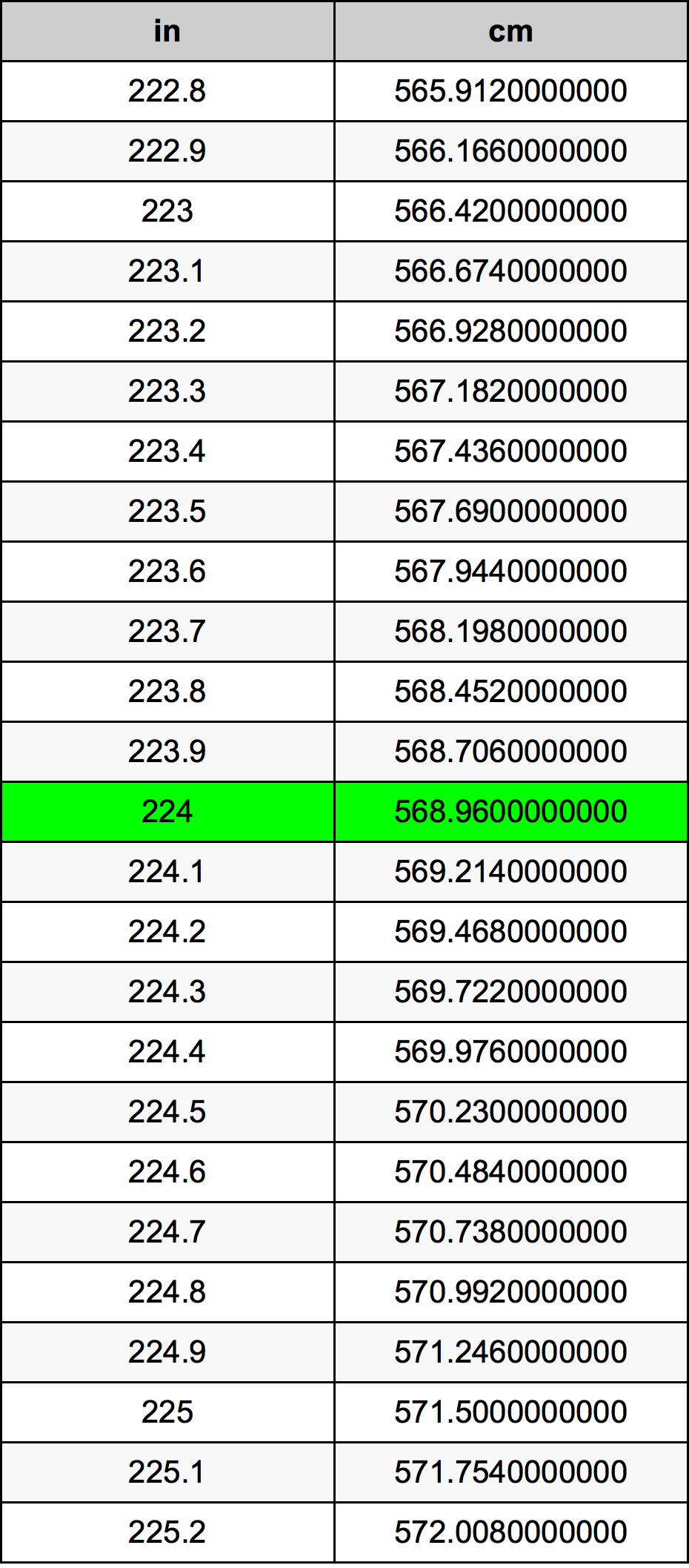Inches To Centimeters

# 224 in to cm224 Inches to Centimeters

in
=
cm

## How to convert 224 inches to centimeters?

 224 in * 2.54 cm = 568.96 cm 1 in
A common question is How many inch in 224 centimeter? And the answer is 88.188976378 in in 224 cm. Likewise the question how many centimeter in 224 inch has the answer of 568.96 cm in 224 in.

## How much are 224 inches in centimeters?

224 inches equal 568.96 centimeters (224in = 568.96cm). Converting 224 in to cm is easy. Simply use our calculator above, or apply the formula to change the length 224 in to cm.

## Convert 224 in to common lengths

UnitLength
Nanometer5689600000.0 nm
Micrometer5689600.0 µm
Millimeter5689.6 mm
Centimeter568.96 cm
Inch224.0 in
Foot18.6666666667 ft
Yard6.2222222222 yd
Meter5.6896 m
Kilometer0.0056896 km
Mile0.0035353535 mi
Nautical mile0.0030721382 nmi

## What is 224 inches in cm?

To convert 224 in to cm multiply the length in inches by 2.54. The 224 in in cm formula is [cm] = 224 * 2.54. Thus, for 224 inches in centimeter we get 568.96 cm.

## 224 Inch Conversion Table## Alternative spelling

224 Inches to cm, 224 Inches in cm, 224 Inch to Centimeters, 224 Inch in Centimeters, 224 in to Centimeters, 224 in in Centimeters, 224 Inch to Centimeter, 224 Inch in Centimeter, 224 Inch to cm, 224 Inch in cm, 224 in to cm, 224 in in cm, 224 Inches to Centimeter, 224 Inches in Centimeter